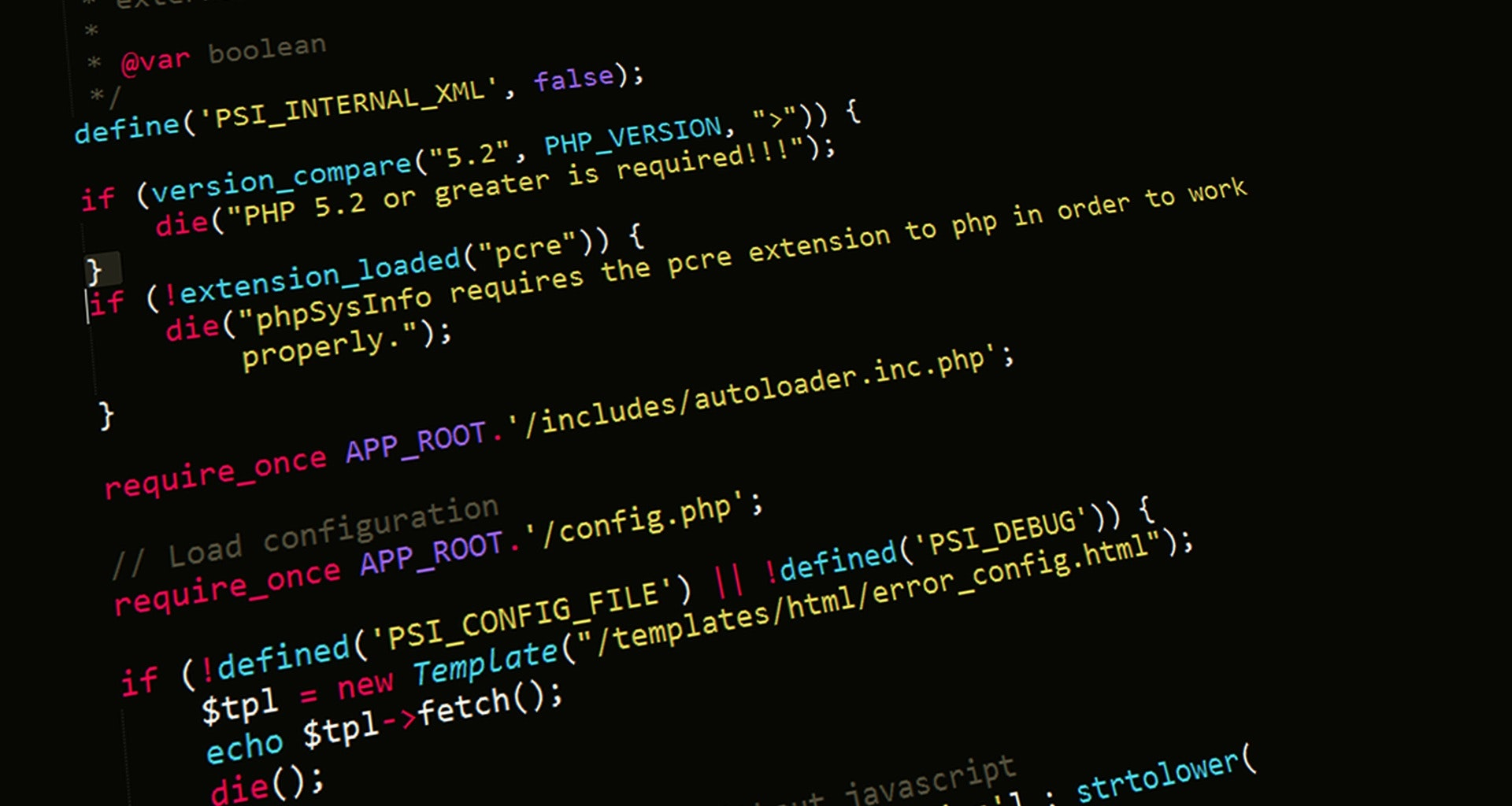Nitish Kumar Singh4 minutes
Posted on
February 2, 2020

# Creating Simple Calculator using PHP

If you are visual learner and prefer video tutorial then here you go.

There is possibility that playing this is at your current location is not approcriate then you can go through the article and you will get the same knowledge.

## Introduction #

In this article we are going to learn about creating Simple Calculator using PHP. Simple Calculator means a calculator which is capable of doing simple operation like add, subtract, product (multiply) and division.

## Prerequistes #

1. Understanding of basic HTML
2. php installation
3. basic php knowledge

## Get Started #

1. Create One folder `learn`( you can name folder anything ).
2. Open the folder in your editor and create one new file `index.php`.

As I told you our calculator is capable of doing only three jobs(tasks).

• Subtraction
• Multiplication
• Division

We require atleast two number to perform any of these operations. We will crete two input field for getting user input. We will require four different button of four different operations.

We are going to write all the things in one file. We don’t require multiple file to do build this calculator.

## Creting UI for Calculator #

 `````` 1 2 3 4 5 6 7 8 9 10 11 12 13 14 15 16 17 18 19 20 21 22 23 24 25 26 27 28 29 30 31 32 33 34 35 36 37 38 `````` `````` PHP - Calculator
``````

If you will see the output of the above image then you will find that you have a simple calculator with 3 input box and four button.

## Let’s come to php #

Now we need to write php for making the calculor working

 `````` 1 2 3 4 5 6 7 8 9 10 11 12 13 14 15 16 17 18 19 20 21 22 23 24 25 26 27 28 29 30 31 `````` `````` ``````

## Learning #

Here are the things which you might you have learned from this article.

• switch : The switch statement is similar to a series of IF statements on the same expression.
• \$_GET `(PHP Global Variables)` : This is used to get data from the url.
• isset : It checks if the variable is `defined` and also not `NULL`.
• is_numeric : Finds whether a variable is a `number` and not other than that.

## Final Code #

If you want the complete code and want to run in locally in your computer then here you go.

 `````` 1 2 3 4 5 6 7 8 9 10 11 12 13 14 15 16 17 18 19 20 21 22 23 24 25 26 27 28 29 30 31 32 33 34 35 36 37 38 39 40 41 42 43 44 45 46 47 48 49 50 51 52 53 54 55 56 57 58 59 60 61 `````` `````` Question 1

PHP - Simple Calculator Program

" method="GET">
">
">
``````
Comment Box